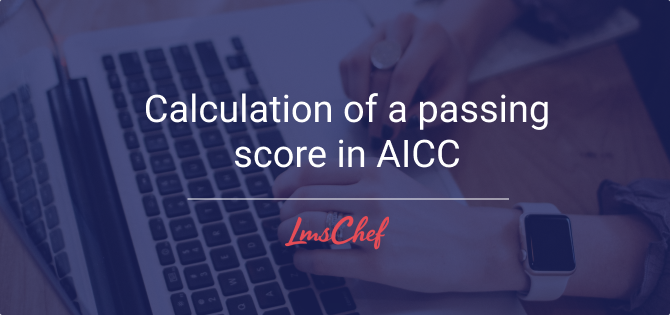# Calculation of a passing score in AICCIn this article, you’ll learn what AICC is about, what its place is in today’s e-learning and, most importantly, how to calculate an AICC passing score. Let’s get started.

## What is AICC?

Just like SCORM or xAPI, AICC is a data model that allows for things like Learning Management Systems (or LMSs) and online training content to exist. When AICC “rules” are implemented in both an LMS and learning content, it enables the LMS to record all learner activity on this content piece.
And just like SCORM, it’s very old. In fact, AICC was the very first truly popular e-learning standard, and it helped us move towards interoperability. So, its importance can’t be underestimated. In 2014, the very organization that created the standard disbanded, and left AICC without support. Luckily, most of the members of this organization moved over to the cmi5 project that would become the next step in e-learning evolution.
If you’d like to read more about the history of AICC, their website has been down since 2015, but fortunately, it is still available on the Way Back Machine. Also, there’s a great article about the history of e-learning standards, including AICC, on scorm.com.

### Here’s how AICC is different from SCORM:

The latter only allows the use of the Javascript API method of communication between LMS, content, and learner. AICC also supports HACP (HTTP communication protocol), which made the communication between LMS content and learner more secure and also enabled the content to be stored on a separate server from where the LMS is hosted. However, both eLearning formats allow the tracking of learner activity and count the scores.

## How is a Passing Score Calculated in AICC?

The CMI model that was first introduced with AICC features a few score types that can be passed from a learning module to an LMS. Note that the following parameters can only have numeric values that usually represent some kind of an abstract point-based system:

• Cmi.score.raw – the number of points a student has at the current point of progress in an e-learning module. This is measured against the student data.mastery score to determine whether the module has been passed or failed.
• Cmi.score.max – the maximum number of points available for completing all interactions in a module. Score.raw should always be lesser or equal to this value.
• Cmi.score.min – the minimum of possible points for a module. Score.raw should always be bigger or equal to this value.
• Student data.mastery score – this is the passing score that is hardcoded into the learning module by its creator. It’s compared to the core.score.raw value to learn if the passing criteria for the module were met or not.

So, the student data.mastery score parameter is what we are actually looking for. But, how is it calculated? Well, it depends on the authoring tool that was used to create our learning module so, this would be the first answer to the main question of this article:

“The way you calculate a passing score in AICC depends on the authoring tool that was used to create the AICC module.”

Still, there are some common principles in how learning modules are built, no matter what authoring tool was used to produce them. In a generic learning module, there’s usually a slide segment that contains theoretical information and various media, followed by a quiz segment that’s supposed to demonstrate how well learners have mastered the theoretical part.

So, if we take a hypothetical module that contains a theoretical part and a quiz, here’s a simple explanation of how a total passing score would be calculated for it:

“Calculate the arithmetic average of all the passing scores in the module.”

Here’s a detailed explanation:

P = (T + Q)/2

Where:

• P – total passing score for the module.
• T – passing score for the theoretical part. It’s calculated like this: take the number of slides with the theory you need to view to get the pass grade and divide them by the total number of slides.
• Q – passing score for the quiz. Number of points acquired in the quiz divided by the maximum number of points you can score in the quiz.

If there are two quizzes in the module, the formula will look like this:

P = (T + Q1 + Q2)/3

Where:

• Q1 – quiz #1
• Q2 – quiz #2

SCORM is really similar to AICC because it was built using the same CMI data model that AICC created, so the same rules would apply to SCORM too.

## Final Words

This is how an AICC passing score is calculated for a learning module. If you have any questions on the topic of this article or anything related to AICC or SCORM, please feel free to let me know in the comments.

Rating
( 1 assessment, average 5 from 5 )1.1.